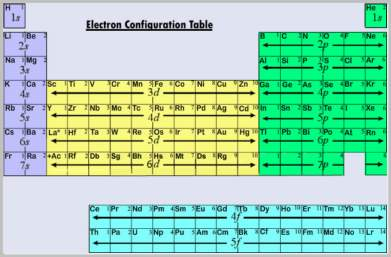# What is an Electron Configuration Chart

The essence of chemistry is the redistribution of atomic valence electrons.  This redistribution lowers the total energy of the atoms, thereby driving chemical reactions.  The electronic configuration of an atom completely specifies the properties of all the electrons.  Knowing the properties of an element’s electrons reveals a great deal about the chemical reactivity of that element.  This information is neatly summarized in the electron configuration chart, which provides the knowledgeable user with ground-state and excited-state electron configurations for most elements.

Electrons live in atomic orbitals, and their properties are described by an ‘address’ consisting of four quantum numbers.  First is the principal quantum number n.  N is a positive integer that identifies a shell containing orbitals having the same energy.  The energy increases as n increases.

Second, the angular momentum quantum number λ, where λ is any integer satisfying 0 ≤ λ ≤ (n-1), identifies subshells containing orbitals with the same orbital angular momentum.  The shape of the orbitals is determined by λ.  An s orbital (λ = 0) is spherically symmetric about the atom’s nucleus, a p orbital (λ = 1) has 2 opposed lobes, a d orbital (λ = 2) looks like a 3-d cloverleaf, and the f orbital (λ = 3) resembles a pair of baby pacifiers connected back-to-back.  G and higher orbitals can be encountered in sufficiently large atoms, but are mainly a complication here.

The magnetic quantum numberis an integer -λ ≤ m ≤ λ that describes the orientation of the orbitals.  The s subshell has no angular momentum, so only one orientation is possible.  As a result, the s subshell contains only a single s orbital.  More orbitals can be accommodated as λ increases, so a p subshell contains 3 p orbitals, the d subshell contains 5 d orbitals, and the f subshell has 7 f orbitals.

Finally, the Pauli Exclusion Principle tells us that each orbital can accommodate no more than two electrons, which are called spin-up (↑) or spin-down (↓).  As 2 electrons can fit into each orbital, we say that an s subshell can hold 2 electrons, a p subshell can hold 6 electrons, a d subshell holds 10 electrons, and an f subshell holds up to 14.  The quantum numbers n, λ, m, and ↑↓ together completely specify the location and properties of an electron belonging to an atom.Using conventional notation, the electronic configuration of an atom is given by describing how many electrons are in each subshell of orbitals.  Consider an isolated atom of sodium in the ground state.  2 electrons are in the lowest energy s subshell, 2 electrons are in the second lowest energy s subshell, 6 electrons are in the lowest energy p subshell (recall that there is no p subshell in the lowest energy shell), and 1 electron is in the third lowest energy s subshell.  This electronic configuration is written as 1s2 2s2 2p6 3s1.  Another way of expressing this electronic configuration is [Ne] 3s1, meaning that the core electrons are in the same places they occupy in a neon atom, and there is one valence electron located in the 3s orbital.

There are rules about which orbitals are occupied by the electrons of a given atom in the ground state.  The electron configuration chart below summarizes the first two of Hund’s rules and the aufbau principle, which says that low energy orbitals are filled before electrons can be placed in higher energy orbitals.  Although not universally true, this is a sufficiently good approximation for us to consider it a rule which has a few exceptions.

λ = 0            1             2            3

2            6            10          14     electrons which can occupy an orbital

n=1     1s

n=2     2s          2p

n=3     3s          3p          3d

n=4     4s          4p          4d          4f

n=5     5s          5p          5d          5f

n=6     6s          6p          6d

n=7     7s          7p

The order in which orbitals are filled is given by moving down diagonal lines radiating upward and right from each of the s orbitals.  The first such line only contains the 1s orbital, so that is filled first.  The second line contains the 2s orbital, which is filled second.  The third line contains the 2p and 3s orbitals.  Moving down the line tells us that the 2p orbitals are filled before the 3s orbitals, and so on.  This chart describes the electron configuration of elements up to an atomic number of 118, but it will give questionable results on the heaviest elements due to relativistic effects.

As an example, titanium has 22 electrons (the atomic number is 22).  The electron configuration of titanium according to the electron configuration chart is 1s2 2s2 2p6 3s2 3p6 4s2 3d2.

The electron configuration of some atoms does not follow this simple set of rules.  For example, the electronic configuration of chromium (atomic number 24) is not 1s2 2s2 2p6 3s2 3p6 4s2 3d4, but rather 1s2 2s2 2p6 3s2 3p6 4s1 3d5, that is, an electron has been promoted from the 4s orbital to a 3d orbital.  This is an example of Hund’s third rule, which states that an electron will be promoted from the nearest s orbital to form a half-filled or completely filled orbital.  In the case of chromium, promoting an electron from 4s to 3d causes both 4s and 3d to be half-filled (1 and 5 electrons, respectively), which lowers the energy of the atom

When one is familiar with both atomic structure and the electron configuration chart, a great deal of chemistry is explainable without the need for lengthy calculations. This chart is one of the most valuable tools for the clever chemist…